# Singular Point

Also found in: Dictionary, Thesaurus, Wikipedia.

## singular point

[′siŋ·gyə·lər ′pȯint]
(mathematics)
For a differential equation, a point that is a singularity for at least one of the known functions appearing in the equation.
A point on a curve at which the curve possesses no smoothly turning tangent, or crosses or touches itself, or has a cusp or isolated point.
A point on a surface whose coordinates, x, y, and z, depend on the parameters u and v, at which the Jacobians D (x,y)/ D (u,v), D (y,z)/ D (u,v), and D (z,x)/ D (u,v) all vanish.

## Singular Point

(in some contexts singularity, critical point).

Singular point of a curve. A singular point of the curve defined’ by the equation F (x, y) = 0 is a point M0 (x0, y0) at which both partial derivatives of the function F (x, y) vanish: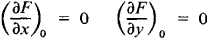If the second partial derivatives of F(x, y) are not all equal to zero at M0, then M0 is called a double point. If all the first and second derivatives vanish at M0, but the third derivatives are not all equal to zero, then M0 is called a triple point, and so on.

When the nature of a curve near a double point is investigated, an important role is played by the sign of the expression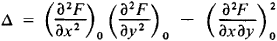If ∆ > 0, the singular point is isolated. Thus the origin is an isolated singular point of the curve y2x4 + 4x2 = 0 (see Figure 1).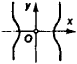Figure 1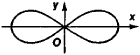Figure 2

If ∆ < 0, the singular point is a node, or a point of self-intersection. For example, the origin is a node of the curve (x2 + y2 + a2)2 – 4a2x2a4 = 0 (see Figure 2). If ∆ = 0, either the singular point is isolated or the branches of the curve have a common tangent at it. Examples of the second case include (1) a cusp of the first kind, where the branches of the curve are on opposite sides of the common tangent and form a cusp, as in the curve y2x3 = 0 (see Figure 3, a); (2) a cusp of the second kind, where the branches of the curve are on the same side of the common tangent, as in the curve (yx2)2x2 = 0 (see Figure 3, b); and (3) a point of osculation—in the curve y2x2 = 0 the origin is a point of osculation (see Figure 3, c).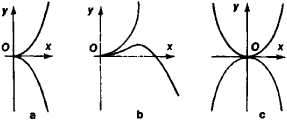Figure 3

Many other types of singular points also have special names, for example, an asymptotic point, which is the vertex of a spiral with infinitely many turns (see Figure 4), and a corner point.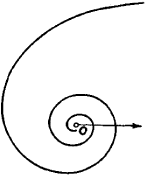Figure 4

Singular point of a differential equation (in English usually critical point). A critical point of the differential equation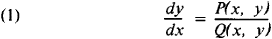where P and Q are continuously differentiable functions, is a point at which the numerator and denominator of the right-hand side of the equation vanish simultaneously. Assuming the critical point to be located at the origin, we can use Taylor series to put equation (1) into the form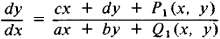where P1 (x, y) and Q1 (x, y) are infinitesimal compared to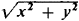The behavior of the integral curves near a critical point depends on the roots λ1 and λ2 of the characteristic equation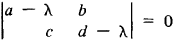Specifically, if λ1 ≠ λ2 and λ1λ2 > 0 or λ1 = λ2, then the critical point is a node; all integral curves in a sufficiently small neighborhood of the node enter the node. If λ1 ≠ λ2 and λ1 λ2 < 0, the critical point is a saddle point; in a neighborhood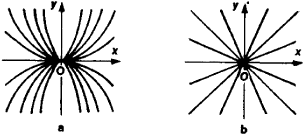Figure 5

of a saddle point four half-line integral curves enter the critical point. Between the four half-lines there are four regions, and each contains a family of integral curves resembling hyperbolas. If λ1,2 = α ± i β, where α ≠ 0 and β ≠ 0, then the critical point is a focus; all integral curves in a sufficiently small neighborhood of a focus are spirals with an infinite number of turns in an arbitrarily small neighborhood of the focus. If, finally, λ1,2 = ±iβ, where β ≠ 0, the nature of the critical point cannot be determined from just the linear terms in the expansions of P(x, y) and Q(x, y); the critical point may be a focus or a center, or it may have an even more complicated nature. In the neighborhood of a center all integral curves are closed and enclose the center. Thus, the point (0, 0) is a node for the equations y′ = 2y/x, where λ1 = 1 and λ2 = 2 (see Figure 5, a), and y′ = y/x, where λ1 = λ2 = 1 (see Figure 5, b). It is a saddle point for the equation y′ = –y/x, where λ1 = –1 and λ2 = 1 (see Figure 6). It is a focus for the equation y′ = (x + y)/(x – y), where λ1 = 1 – i and λ2 = 1 + i (see Figure 7). Finally, it is a center for the equation y′ = – x/y, where λ1 = – i and λ2 = i (see Figure 8).

If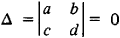then the critical point is called a critical point of higher order. A critical point of higher order can belong to one of the types indicated above, or it can be of an even more complicated nature. When the functions P(x, y) and Q(x, y) are analytic, a neighborhood of a critical point of higher order can split into elliptic (D1), parabolic (D2), and hyperbolic (D3) regions (see Figure 9). Elliptic regions are occupied by integral curves both ends of which enter the critical point; parabolic regions are occupied by integral curves one end of which enters the critical point; and hyperbolic regions are bounded by two integral curves that enter the critical point—the regions are occupied by integral curves that resemble hyperbolas and are located between the bounding integral curves. If no integral curves enter the critical point, then the critical point is said to be stable. A neighborhood of a stable critical point consists of closed integral curves enclosing the critical point with spirals located between the curves (see Figure 10).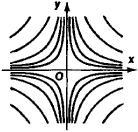Figure 6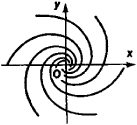Figure 7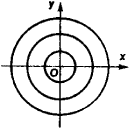Figure 8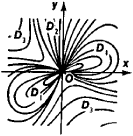Figure 9

The study of the critical points of differential equations is essentially the study of the behavior of families of integral curves in the neighborhoods of the critical points. It is a branch of the qualitative theory of differential equations and plays an important role in applications, especially in problems of the stability of motion. The works of A. M. Liapunov, H. Poincaré, and others are important in this connection.

Singular point of a single-valued analytic function (in English usually singularity). A singularity of a single-valued analytic function is a point at which the function’s analyticity is violated. If there exists a neighborhood of a singularity a free of other singularities, then a is called an isolated singularity. If a is an isolated singularity and if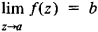exists and is finite, then a is called a removable singularity. By making a suitable change in the definition of the function at a – namely, f(a) = b – or by extending the definition to this point if the function is not defined there, we can make a into a point of analyticity of the function. For example. since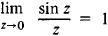the point z = 0 is a removable singularity of the function f(z) = (sin z)/z and a point of analyticity of the function f1 (z), with f1 (z) = f(z) for z ≠ 0 and f1 (0) = 1. If a is an isolated singularity of f(z) and the limit of f(z) and z approaches a is infinity, then a is called a pole of f(z). If the limit of f(z) as z approaches a does not exist, a is called an essential singularity of f(z). The Laurent series of f(z) about a may contain no negative powers of za, a finite number of such powers, or an infinite number of such powers. Accordingly, a is said to be a removable singularity, a pole, or an essential singularity of f (z). If a is a pole of f (z), then its order is the highest power of l/(za) in the Laurent series of f(z) about a. For example, z = 0 is a pole of order p of the function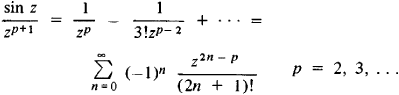and an essential singularity of the function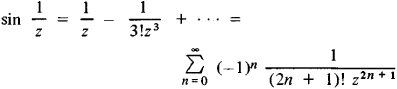On the circumference of the circle of convergence of a power series there must be at least one singularity of the function represented inside the circle by the given power series. All boundary points of the existence dòmain of a single-valued analytic function (the natural boundary) are singular points of this function. Thus, all points on the unit circle ǀzǀ = 1 are singular with respect to the function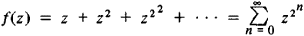The concept of a singularity is more complicated for multiple-valued functions. Besides the singularities in the individual sheets of the Riemann surface of a function, that is, the singularities of the single-valued branches, every branch point is also a singularity of the function. An isolated branch point of a Riemann surface is a branch point such that in some neighborhood of the point there are no other singularities of the function on any of the sheets. There are several types of such branch points. If a is an isolated branch point of finite order and the limit of f(z) as z approaches a exists and is finite, then the singularity is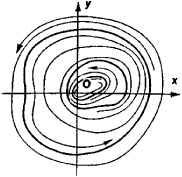Figure 10

called an ordinary critical point. But if the limit is infinite, a is called a critical pole. If a is an isolated branch point of infinite order and the limit (finite or infinite) of f(z) as z approaches a exists, then a is called a transcendental singularity. All other isolated branch points are called critical essential singularities. For example, the point z = 0 is an ordinary critical point for the function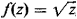, a critical pole of the function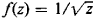, a transcendental singularity of the function f(z) = 1n z, and a critical essential singularity of the function f(z) = sin 1n z.

Every nonremovable singularity blocks analytic continuation; that is, analytic continuation along a curve passing through a nonremovable singularity is impossible.

## Singular Point

A singular point on a phase diagram or a composition-property diagram is a point that corresponds to the formation of a nondissociated compound. For example, in a system of two components A and B, the formation of such a compound C is expressed by the point D (seeBINARY SYSTEMS). This point is the intersection of two branches of the liquidus curve (the geometric locus of the temperatures at which crystallization begins), which belong to a single continuous curve corresponding to the formation of the solid phase C from the liquid, as required by the principles of continuity and correspondence. Singular points are observed on the composition-property diagrams of liquid systems and solid solutions if transformations occur that are accompanied by the formation of certain daltonides (seeDALTONIDES AND BERTHOLLIDES).

### REFERENCES

Kurnakov, N. S. Izbr. trudy, vols. 1-3. Moscow, 1960-63.
Anosov, V. Ia., and S. A. Pogodin. Osnovnye nachala fiziko-khimicheskogo analiza. Moscow-Leningrad, 1947. [23–1230–]
References in periodicals archive ?
The corner points of the strip and singular points of the type E are sources of the perturbation, causing both longitudinal (regions c, e and g) and transverse (regions b, d and g) waves.
To eliminate the singular points in the original data, a statistics-based analysis method is applied, which is characterized by its simple structure, small calculating amount, and fast speed .
The general Heun equation [1-3], which is the most general second-order linear ordinary differential equation having four regular singular points, is currently widely encountered in physics and mathematics research (see, e.g., [1-14] and references therein).
More precisely we show that, in this case, a neighborhood of each singular point with j = 1, ..., 6, can be identified with a cone over a manifold, called the link of m.
The bilateral filtering phase recovers singular points and removes artifacts on silhouettes by averaging depth data with restrictions of both depth difference and RGB similarity.
Thus, we can span the "normalized slow dynamics" on the tangent bundle at the critical manifold [M.sub.0] at the pseudo singular point. This leads to the so-called desingularized vector field:
For instance, any closed phase curve of such a vector field is convex and contains no more than one singular point inside.
--Careful examination of (3) reveals the fact that there exists a singular point at [[kappa].sub.1] = 0, that is at [k.sub.[rho]] = [k.sub.01] and hence it must be excluded by a sufficient range around [k.sub.01], for the numerical integration algorithm to converge.
Siddharth's singular point of view also shines through: He has a penchant for pave-set diamonds and unconventional stone shapes, like cabochons and briolettes--all atypical of Indian jewelry conventions.
"Pomoderna and seven monochroms- thus rejected the idea of a singular point of view or an authentic and true image.

Site: Follow: Share:
Open / Close